# gammatoneFilterBank

Gammatone filter bank

Since R2019a

## Description

`gammatoneFilterBank` decomposes a signal by passing it through a bank of gammatone filters equally spaced on the ERB scale. Gammatone filter banks were designed to model the human auditory system.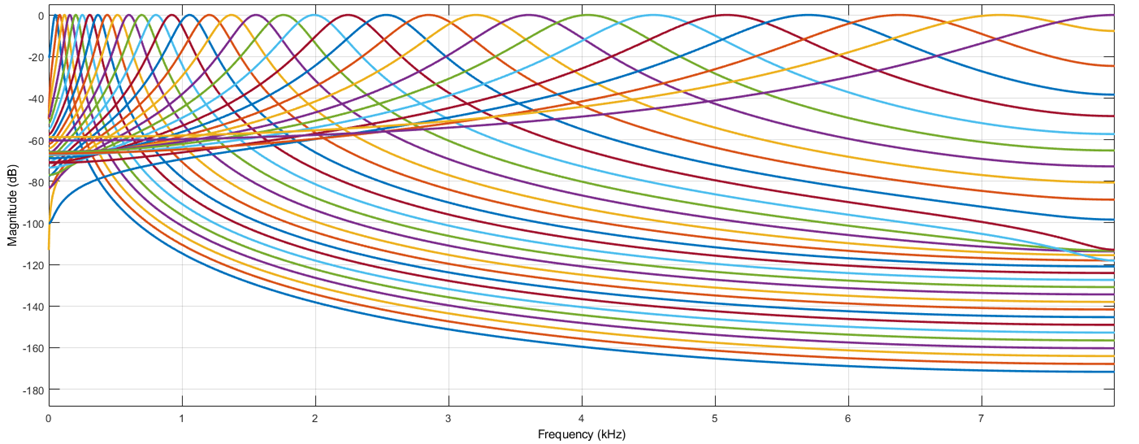To model the human auditory system:

1. Create the `gammatoneFilterBank` object and set its properties.

2. Call the object with arguments, as if it were a function.

## Creation

### Syntax

``gammaFiltBank = gammatoneFilterBank``
``gammaFiltBank = gammatoneFilterBank(range)``
``gammaFiltBank = gammatoneFilterBank(range,numFilts)``
``gammaFiltBank = gammatoneFilterBank(range,numFilts,fs)``
``gammaFiltBank = gammatoneFilterBank(___,Name,Value)``

### Description

````gammaFiltBank = gammatoneFilterBank` returns a gammatone filter bank. The object filters data independently across each input channel over time.```
````gammaFiltBank = gammatoneFilterBank(range)` sets the `Range` property to `range`.```
````gammaFiltBank = gammatoneFilterBank(range,numFilts)` sets the `NumFilters` property to `numFilts`.```
````gammaFiltBank = gammatoneFilterBank(range,numFilts,fs)` sets the `SampleRate` property to `fs`.```
````gammaFiltBank = gammatoneFilterBank(___,Name,Value)` sets each property Name to the specified Value. Unspecified properties have default values.Example: ```gammaFiltBank = gammatoneFilterBank([62.5,12e3],'SampleRate',24e3)``` creates a gammatone filter bank, `gammaFiltBank`, with bandpass filters placed between 62.5 Hz and 12 kHz. `gammaFiltBank` operates at a sample rate of 24 kHz.```

## Properties

expand all

Unless otherwise indicated, properties are nontunable, which means you cannot change their values after calling the object. Objects lock when you call them, and the `release` function unlocks them.

If a property is tunable, you can change its value at any time.

Frequency range of the filter bank in Hz, specified as a two-element row vector of monotonically increasing values.

Tunable: No

Data Types: `single` | `double` | `int8` | `int16` | `int32` | `int64` | `uint8` | `uint16` | `uint32` | `uint64`

Number of filters in the filter bank, specified as a positive integer scalar.

Tunable: No

Data Types: `single` | `double` | `int8` | `int16` | `int32` | `int64` | `uint8` | `uint16` | `uint32` | `uint64`

Input sample rate in Hz, specified as a positive scalar.

Tunable: Yes

Data Types: `single` | `double`

## Usage

### Syntax

``audioOut = gammaFiltBank(audioIn)``

### Description

example

````audioOut = gammaFiltBank(audioIn)` applies the gammatone filter bank on the input and returns the filtered output.```

### Input Arguments

expand all

Audio input to the filter bank, specified as a scalar, vector, or matrix. If specified as a matrix, the columns are treated as independent audio channels.

Data Types: `single` | `double`

### Output Arguments

expand all

Audio output from the filter bank, returned as a scalar, vector, matrix, or 3-D array. The shape of `audioOut` depends on the shape of `audioIn` and `NumFilters`. If `audioIn` is an M-by-N matrix, then `audioOut` is returned as an M-by-`NumFilters`-by-N array. If N is 1, then `audioOut` is returned as a matrix.

Data Types: `single` | `double`

## Object Functions

To use an object function, specify the System object™ as the first input argument. For example, to release system resources of a System object named `obj`, use this syntax:

`release(obj)`

expand all

 `fvtool` Visualize filter bank `freqz` Compute frequency response `getCenterFrequencies` Center frequencies of filters `getBandwidths` Get filter bandwidths `coeffs` Get filter coefficients `info` Get filter information
 `step` Run System object algorithm `release` Release resources and allow changes to System object property values and input characteristics `reset` Reset internal states of System object

## Examples

collapse all

Create a default gammatone filter bank for a 16 kHz sample rate.

```fs = 16e3; gammaFiltBank = gammatoneFilterBank(SampleRate=fs) ```
```gammaFiltBank = gammatoneFilterBank with properties: FrequencyRange: [50 8000] NumFilters: 32 SampleRate: 16000 ```

Use `fvtool` to visualize the response of the filter bank.

```fvtool(gammaFiltBank) ```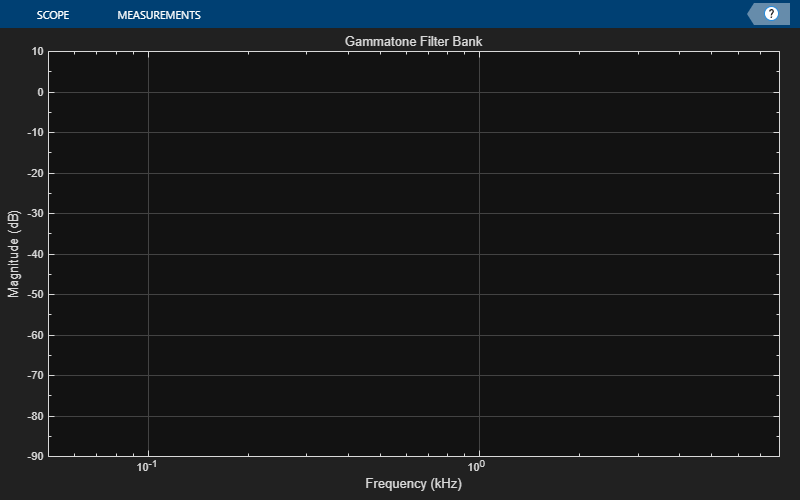Process white Gaussian noise through the filter bank. Use a spectrum analyzer to view the spectrum of the filter outputs.

```sa = spectrumAnalyzer(SampleRate=16e3,... PlotAsTwoSidedSpectrum=false,... FrequencyScale="log"); for i = 1:5000 x = randn(256,1); y = gammaFiltBank(x); sa(y); end ```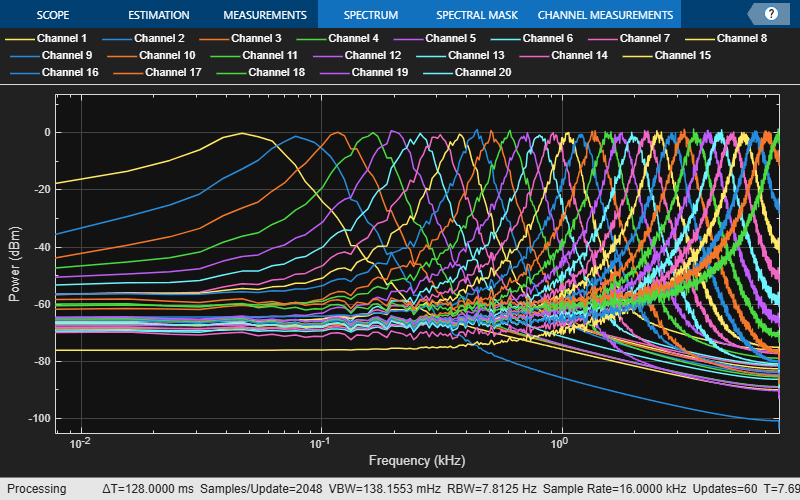This example illustrates a nonoptimal but simple approach to analysis and synthesis using `gammatoneFilterBank`.

Read in an audio file and listen to its contents.

```[audioIn,fs] = audioread('Counting-16-44p1-mono-15secs.wav'); sound(audioIn,fs)```

Create a default `gammatoneFilterBank`. The default frequency range of the filter bank is 50 to 8000 Hz. Frequencies outside of this range are attenuated in the reconstructed signal.

`gammaFiltBank = gammatoneFilterBank('SampleRate',fs)`
```gammaFiltBank = gammatoneFilterBank with properties: FrequencyRange: [50 8000] NumFilters: 32 SampleRate: 44100 ```

Pass the audio signal through the gammatone filter bank. The output is 32 channels, where the number of channels is set by the `NumFilters` property of the `gammatoneFilterBank`.

```audioOut = gammaFiltBank(audioIn); [N,numChannels] = size(audioOut)```
```N = 685056 ```
```numChannels = 32 ```

To reconstruct the original signal, sum the channels. Listen to the result.

```reconstructedSignal = sum(audioOut,2); sound(reconstructedSignal,fs)```

The gammatone filter bank introduced various group delays for the output channels, which results in poor reconstruction. To compensate for the group delay, remove the beginning delay from the individual channels and zero-pad the ends of the channels. Use `info` to get the group delays. Listen to the group delay-compensated reconstruction.

```infoStruct = info(gammaFiltBank); groupDelay = round(infoStruct.GroupDelays); % round for simplicity audioPadded = [audioOut;zeros(max(groupDelay),gammaFiltBank.NumFilters)]; for i = 1:gammaFiltBank.NumFilters audioOut(:,i) = audioPadded(groupDelay(i)+1:N+groupDelay(i),i); end reconstructedSignal = sum(audioOut,2); sound(reconstructedSignal,fs)```

Read in an audio signal and convert it to mono for easy visualization.

```[audio,fs] = audioread('WaveGuideLoopOne-24-96-stereo-10secs.aif'); audio = mean(audio,2);```

Create a `gammatoneFilterBank` with 64 filters that span the range 62.5 to 20,000 Hz. Pass the audio signal through the filter bank.

```gammaFiltBank = gammatoneFilterBank('SampleRate',fs, ... 'NumFilters',64, ... 'FrequencyRange',[62.5,20e3]); audioOut = gammaFiltBank(audio);```

Calculate the energy-per-band using 50 ms windows with 25 ms overlap. Use `dsp.AsyncBuffer` to divide the signals into overlapped windows and then to log the RMS value of each window for each channel.

```samplesPerFrame = round(0.05*fs); samplesOverlap = round(0.025*fs); buff = dsp.AsyncBuffer(numel(audio)); write(buff,audioOut.^2); sink = dsp.AsyncBuffer(numel(audio)); while buff.NumUnreadSamples > 0 currentFrame = read(buff,samplesPerFrame,samplesOverlap); write(sink,mean(currentFrame,1)); end```

Convert the energy values to dB. Plot the energy-per-band over time.

```gammatoneSpec = read(sink); D = 20*log10(gammatoneSpec'); timeVector = ((samplesPerFrame-samplesOverlap)/fs)*(0:size(D,2)-1); cf = getCenterFrequencies(gammaFiltBank)./1e3; surf(timeVector,cf,D,'EdgeColor','none') axis([timeVector(1) timeVector(end) cf(1) cf(end)]) view([0 90]) caxis([-150,-60]) colorbar xlabel('Time (s)') ylabel('Frequency (kHz)')```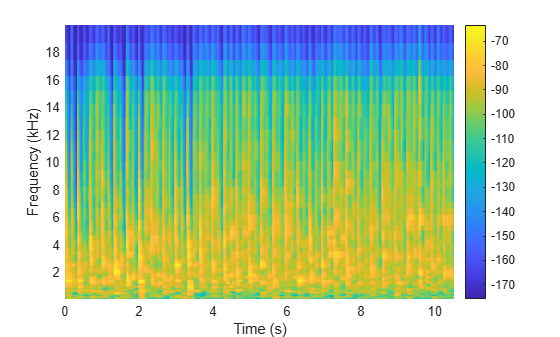## Algorithms

expand all

A gammatone filter bank is often used as the front end of a cochlea simulation, which transforms complex sounds into a multichannel activity pattern like that observed in the auditory nerve. The `gammatoneFilterBank` follows the algorithm described in . The algorithm is an implementation of an idea proposed in . The design of the gammatone filter bank can be described in two parts: the filter shape (gammatone) and the frequency scale. The equivalent rectangular bandwidth (ERB) scale defines the relative spacing and bandwidth of the gammatone filters. The derivation of the ERB scale also provides an estimate of the auditory filter response which closely resembles the gammatone filter.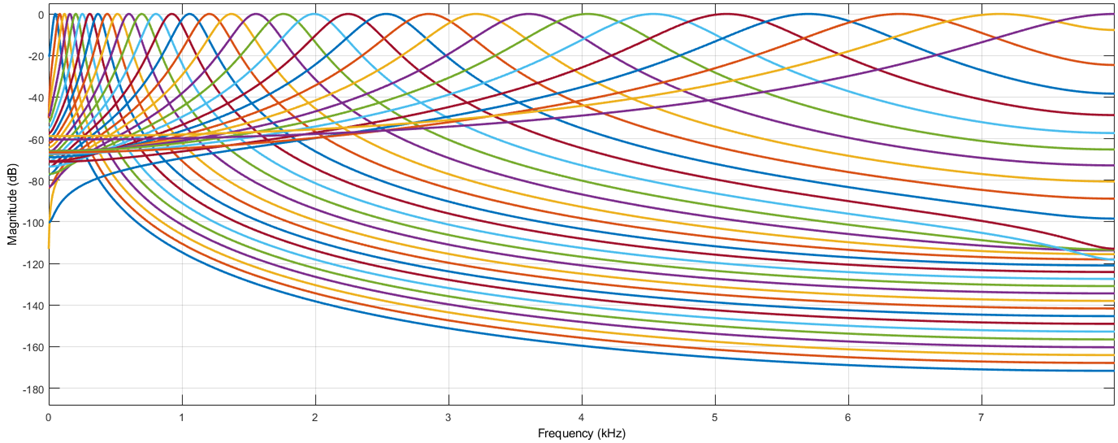Slaney, Malcolm. "An Efficient Implementation of the Patterson-Holdsworth Auditory Filter Bank." Apple Computer Technical Report 35, 1993.

 Patterson, R. D., K. Robinson, J. Holdsworth, D. McKeown, C. Zhang, and M. Allerhand. "Complex Sounds and Auditory Images." Auditory Physiology and Perception. 1992, pp. 429–446.

 Aertsen, A. M. H. J., and P. I. M. Johannesma. "Spectro-temporal Receptive Fields of Auditory Neurons in the Grassfrog." Biological Cybernetics. Vol. 38, Issue 4, 1980, pp. 223–234.

 Glasberg, Brian R., and Brian C. J. Moore. "Derivation of Auditory Filter Shapes from Notched-Noise Data." Hearing Research. Vol. 47. Issue 1-2, 1990, pp. 103 –138.